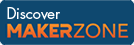Cody

# Problem 1658. Simple equation: Annual salary

Given an hourly wage, compute an annual salary by multiplying the wage times 40 and times 50, because salary = wage x 40 hours/week x 50 weeks/year. The multiplication operator is *.

### Solution Stats

82.42% Correct | 17.58% Incorrect
Last solution submitted on Mar 18, 2019

#### TagsMATLAB and Simulink resources for Arduino, LEGO, and Raspberry Pi